# Electric Field Strength or Electric Field Intensity

The force acting on a unit positive charge inside an electric field is termed as electric field strength or electric field intensity.

Electric field strength or electric field intensity is the synonym of electric field. Electric field strength can be determined by Coulomb’s law. According to this law, the force ‘F’ between two point charges having charge Q1 and Q2 Coulombs and placed at a distance d meter from each other is given by,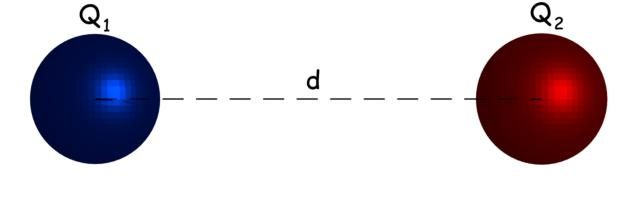Here, εo is the permittivity of vacuum = 8.854 × 10 – 12 F/m and εr is the relative permittivity of the surrounding medium.

Now, let us put Q2 = + 1 Coulomb and let us denote force F by E in the equation (1), and by doing these we get,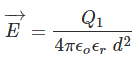This equation shows the force acting the a unit positive charge placed at a distance d from charge Q1.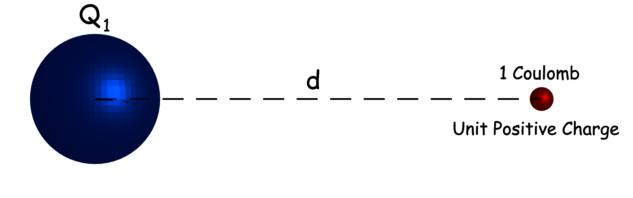As per definition, this is nothing but of electric field strength of charge Q1 at a distance d from that charge. Now, we got the expression of electric field strength or intensity. Now, by combining this expression with equation (1), we get,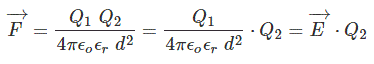The above expression shows that, if we place a charge at any point in an electric field, the product of the electric field strength at that point and the charge of the body gives the force acting on the body at that point in the field. The above expression can also be rewritten as,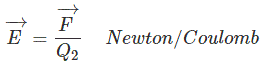Depending on this expression, the electric field strength can be expressed in Newton / Coulomb. That is unit of electric field strength is Newton / Coulomb. The electric field strength has direction and hence it is a vector quantity. Intensity means the magnitude or amount. Now field intensity similarly means the magnitude of the strength of the field. Finally electric field intensity or strength can be written as,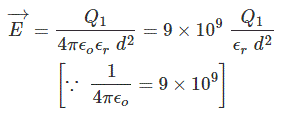So far we have discussed the electric field intensity at a point due to the influence of a single charge, but there may be a case where the point is under the filed of many charged bodies. In that case, we first have to calculate, the electric field strength at that point for individual charges and then we have to vectorially add up all the field strengths to get resultant field strength at that point.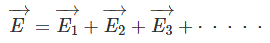Want To Learn Faster? 🎓
Get electrical articles delivered to your inbox every week.
No credit card required—it’s 100% free.